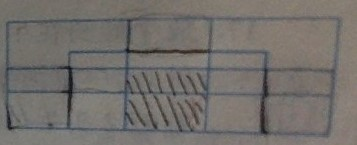•how many rectangles are in here?
This is on a book, and the book's answer is 73, but I count 76.

• Also, what is the last digit of 1^15 + 2^15 + 3^15 +......+ 2011^15 + 2012^15 ?

• PS. for the first question, ignore the stuff I drew, and also, I can assure everyone that the books answer is usually wrong

• Hey @eeveelution ! Sorry for replying so late, but here's how I would look at your two problems:

For the second one, since the problem only cares about the last digit of that huge sum, we only need to look at the last digit of each individual term!

$$1^{15}$$ is easy, the last digit of $$1^{15}$$ is just $$1$$.
For the rest though, we don't want to expand it out (that's a lot of work).

So, for $$2^{15}$$, let's rewrite it as $$(2^5)^3$$.
$$2^5=32 \implies \text{last digit is 2}$$
$$2^3=8$$
So the last digit of $$2^{15}=(2^5)^3$$ is $$8$$.

Another way to look at it is to try and find a pattern among powers of 2. Let's do a few and see if we can find anything.
$$2^1 \implies \text{last digit 2}$$
$$2^2 \implies \text{last digit 4}$$
$$2^3 \implies \text{last digit 8}$$
$$2^4 \implies \text{last digit 6}$$
$$2^5 \implies \text{last digit 2}$$
$$2^6 \implies \text{last digit 4}$$
Wait a minute, this is just gonna repeat $$2,4,8,6,2,4,8,6...$$ It cycles every $$4$$!
So, since $$15$$ is $$3$$ more than a multiple of $$4$$, the last digit of $$2^{15}$$ is going to be the $$3^{\text{rd}}$$ number in the list, which is $$8$$.

You can repeat this process for the rest of the numbers.
But, keep in mind that $$12$$ has units digit $$2$$, so $$12^{15}$$ is going to have the same units digit as $$2^{15}$$!

Also, one last tip-- since $$2012$$ is so big, I would group everything in groups of $$10$$. What I mean is, first find what the units digit of $$1^{15}+2^{15}+...+10^{15}$$ is. Then, since $$11^{15}$$ through $$20^{15}$$ will have the same units digits (along with every other group of 10), you can use a shortcut and not have to calculate every single one.

For the rectangle problem, I'm getting 79... which is weird... but either way I definitely think it's more than 73. What book is this?

• @quacker88 thank you very much! I understand now, and also, for the second one it is a chinese book called 《动脑筋》.